# Math Practice Topic: Circle Area

 Description: This topic covers the concept of finding the area of a circle. Adaptive Learning Progression: First answers use pi, then we use the fraction 22/7 for pi, then we use 3.14 for pi. Start using MathScore for free

## Sample Levels (out of 5)

Find the area. Use 3.14 as an approximation for π.

 1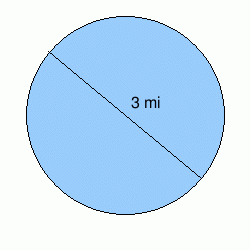Area: 2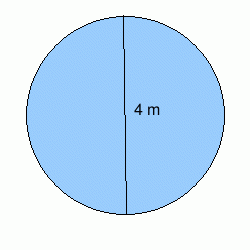Area: 3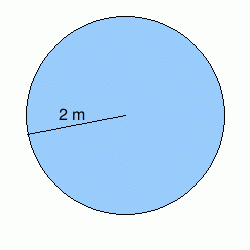Area:

Find the area. Use 3.14 as an approximation for π.

 1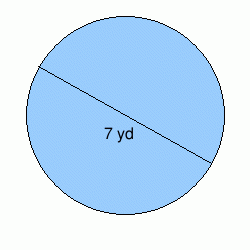Area: 2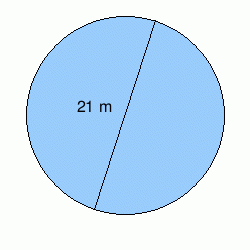Area: 3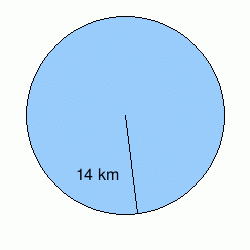Area: 4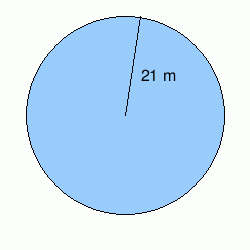Area: 5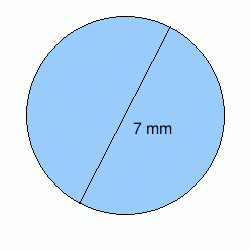Area:

Find the area. Use 3.14 as an approximation for π.

 1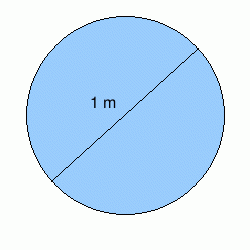Area: 2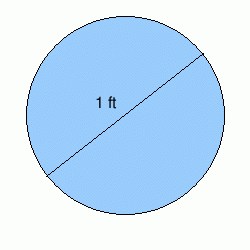Area: 3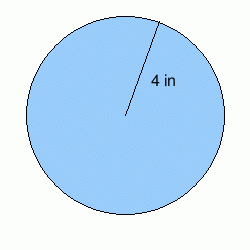Area: